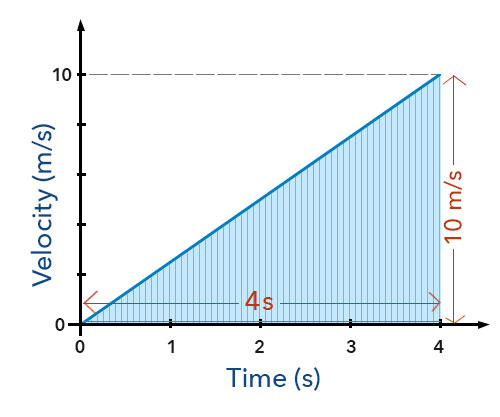## Velocity

Velocity is defined as a measure of the distance an object travels in a stated direction in a given length of time.

Thus velocity is speed in a stated direction. Velocity is referred to as a vector quantity because it possesses both size and direction, the size being speed.

Where speed only tells us how fast or slow an object is moving, it gives no reference of direction, velocity is used as a more complete measure as it not only gives speed but also the direction.

Two cars could be travelling with the same speed of 100 km/h on a motorway. However, by stating one car is travelling 100 km/h north and the other 100 km/h south, do we realise they are travelling in opposite directions, thus the velocity gives a better indication of the motion.

The velocity of an object can be determined from the equation below:

Velocity = Distance travelled in a given direction (meters) Time taken (seconds)

Velocity = Displacement (meters) Time taken (seconds)

### Average Velocity – constant velocity in a straight line

Let us consider an object at a constant velocity travels 50m in a due east direction (with no change to direction) for a duration of 10 seconds.

Here the velocity is in a straight line i.e. due east only. The increase in displacement is 50m and the time taken 10 seconds.

The average velocity for this case can be expressed as:

Average velocity = Increase in displacement (m) Time taken (s)

Average velocity = 50 (m) 10 (s)

Average velocity = 5 m/s due east

### Average Velocity – object accelerating at a constant rate

Let us consider an object takes 4 seconds to accelerate at a constant rate from rest to a velocity of 10 m/s. In this case the displacement is not given however, we know the initial velocity is 0 m/s as the object starts from rest and the final velocity is 10 m/s.

The average velocity for this case can be expressed as:

Average velocity = initial velocity (m/s) + final velocity (m/s) 2

Average velocity = 0 (m/s) + 10 (m/s) 2

Average velocity = 10 (m/s) 2

Average velocity = 5 m/s

The displacement of the object can be determined by multiplying the average velocity with the time.

Displacement = average velocity (m/s) x time (s)

Displacement = 5 (m/s) x 4 (s)

Displacement = 20 m

Below is a velocity-time graph for the objected accelerated at a constant rate:The increasing gradient shows a constant rate acceleration. The area under a velocity time graph is equal to the displacement of the object in a particular time interval (in this case 4 seconds).

Here the area under the graph can be given by the area formula of a triangle:

area = 12 base x height

displacement = 12 time taken x (initial velocity + final velocity)

displacement = 12 x 4 x (0 + 10)

displacement = 12 x 4 x 10

displacement = 12 x 40

displacement = 20 m

It can be seen that this too is equal to the displacement determined from the average velocity.

It can also be shown that the average velocity in this case can be derived from the graph by using the standard equation:

Average velocity = increase in displacement (m) time taken (s)

By inserting the area under the graph for increase in displacement,

Average velocity = 12 time taken x (initial velocity + final velocity) time taken

Cancelling out time taken gives,

Average velocity = 12 time taken x (initial velocity + final velocity) time taken

Average velocity = (initial velocity + final velocity) 2